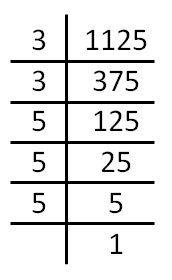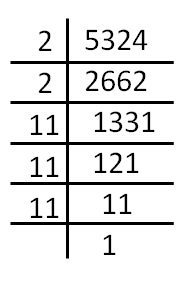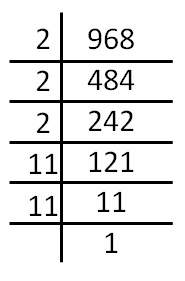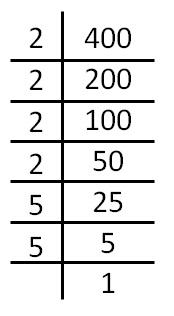Smallest number multiplied to get a perfect cube

Let’s do this by examples

### Finds the smallest number multiplied to 1125 to get a perfect cubeWe see that,

1125  = 3 × 3 × 5 × 5 × 5

Since 3 does not occur triplets,

we multiply by 3 to make a triplet

So, our number becomes

1125 × 3   = 3 × 3 × 5 × 5 × 5 × 3

= 3 × 3 × 3 × 5 × 5 × 5

Now, it becomes a perfect cube.

So, we multiply 1125 by 3 to make it a perfect cube

### Finds the smallest number multiplied to 5324 to get a perfect cube.We see that,

5324 = 2 × 2 × 11 × 11 × 11

Here,

2 does not occur in triplets

So, we multiply by 2 to make triplet

So, our number becomes

5324  × 2 = 2 × 2 × 11 × 11 × 11 × 2

= 2 × 2 × 2 × 11 × 11 × 11

Now, it becomes a perfect cube.

So, we multiply 5324 by 2 to make it a perfect cube

### Finds the smallest number multiplied to 968 to get a perfect cube.We see that,

968 = 2 × 2 × 2 × 11 × 11

Here,

11 does not occur in triplets

So, we multiply by 11 to make triplet

So, our number becomes

968 × 11 = 2 × 2 × 2 × 11 × 11 × 11

Now, it becomes a perfect cube.

So, we multiply 968 by 11 to make it a perfect cube

### Finds the smallest number divided to 400 to get a perfect cube.We see that,

400  = 2 × 2 × 2 × 2 × 5 × 5

Here,

2 & 5 does not occur in triplets

So, we multiply by 2 × 2 × 5 to make triplet

So, our number becomes

400 × 2 × 2 × 5 = 2 × 2 × 2 × 2 × 5 × 5 × 2 × 2 × 5

= 2 × 2 × 2 × 2 × 2 × 2 × 5 × 5 × 5

Now, it becomes a perfect cube.

So, we multiply 400 by 2 × 2 × 5

i.e. 20  to make it a perfect cube

Learn in your speed, with individual attention - Teachoo Maths 1-on-1 Class